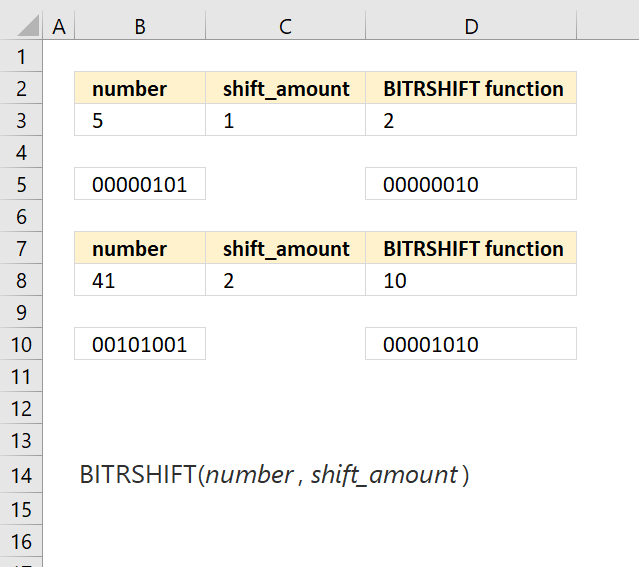Author: Oscar Cronquist Article last updated on May 11, 2022The BITRSHIFT function calculates the number where the binary equivalent is shifted right by a specified number of bits and then converted back to a number.

Formula in cell D3:

=BITRSHIFT(B3, C3)

Number 5 is 00000101 binary. Adding one zero to the left and removing the last digit you get 00000010 binary which is number 2.

### Excel Function Syntax

BITRSHIFT(numbershift_amount)

### Arguments

 number Required. The number you want to shift. shift_amount Required. How many digits you want to shift the binary representation of the number.